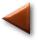## An extremely brief introduction to computational quantum chemistry

In this section, we provide a very brief background for the computational tools to be used in this module, which are based on quantum chemistry. Because quantum theory is not the primary concern of this exercise, important themes in quantum chemistry are addressed in a broad manner. Quantum theory holds that matter has both particle- and wavelike properties. For large particles, the wavelike properties of matter are nearly impossible to detect. However, for small particles—such as electrons—the particle-wave duality must be addressed when writing equations, including energy balance equations. The quantum mechanical energy balance (also known as the Schrödinger equation) for an electron can be written as follows: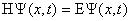(1)

In this equation, E is the total energy of the electron,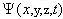psi is the wavefunction for the electron, and H is the Hamiltonian operator that describes the kinetic and potential energy of the electron. In other words, the Schrödinger equation is a simple statement that the total energy is equal to the kinetic plus potential energies for a wavelike particle. The Hamiltonian operator for a single electron can be written as: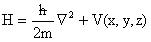(2)

Where V (x,y,z) =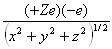(3)

Z is the atomic number, e is the charge associated with an electron, and x,y, and z are spatial components.

And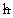is Planck’s constant divided by two Pi, m is the mass of an electron,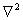is the Laplacian operator, and V(x,y,z) is the potential energy term, which depends on the electrostatic interactions of the electron with nuclei (attractive interactions) and other electrons (repulsive interactions) in the system.

As we will see from the examples in this module, by finding the total energy of molecular systems by solving Schrödinger equations, we can compute thermodynamic and kinetic quantities that are important in chemical reaction engineering (CRE). We start with a solution for the simplest possible molecule – the hydrogen atom, with a single nucleus and single electron – and then show how the results from this solution can be used to address more complex (and relevant) chemical systems.

## The Hydrogen-Like Atom

The Schrödinger equation can only be solved analytically for a “hydrogen-like” atom, i.e., an atom with one electron and one nucleus. The Schrödinger equation is a special type of equation known as an eigenvalue equation, which some of you may have encountered in higher-level mathematics courses. Eigenvalue problems have a number of special characteristics. One of these is that they have an infinite number of solutions for both the eigenvalues (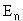) and the associated eigenfunctions(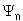). The value n (an integer) is known in quantum mechanics as the principle quantum number. The fact that there are an infinite number of solutions for the energy of our molecule may seem confusing at first, but we will soon see what these solutions for different values of n represent.

The Schrödinger equation—like many other partial differential equations—can be solved using a solution method known as separation of variables subject to the boundary condition that the energy of the system is zero when the electron and nucleus are infinitely far away from each other. We will not go through the details of the solution method here; that method is described in a number of references (click here for references). Instead, we will discuss the form of the solutions to the Schrödinger equation for the hydrogen-like atom.

The lowest energy solution for n=1 describes the state in which the system is most probable to exist; this is also known as the ground state. For n=2 (the next-lowest energy solution) there are four solutions that have equivalent energies; for n=3 (the third-lowest energy solution), the number of energetically equivalent solutions rises to 9. Although the physical meaning of the total energiesfor the system are relatively easy to understand, the meaning of the wavefunction is not. To gain insights into what the wavefunctions actually are, we will consider the quantity: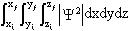(4)

Which is a Cartesian coordinate triple integral over all of the space that the electron could occupy. It can be shown that this quantity describes the probability that the electron will be located in the volume of space between the endpoints labeled i (initial) and f (final). To make this more clear, one can plot the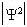probability distribution as a function of position for each of our solutions.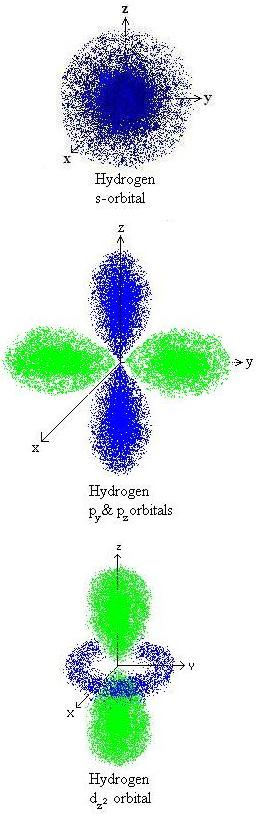We see that our eigenfunctions psiactually describe the electronic orbitals of the hydrogen atoms. We can recognize that the lowest energy solution (for n=1) corresponds to the 1s orbital:which is the orbital that we know should be occupied by an electron in the ground state for an H atom. This is the region in space in which the electron is most probable to be present. For n=2, there are four energetically degenerate wavefunctions that represent the 2s and 2px, 2py, and 2pz orbitals; these are orbitals which are less energetically favorable for occupation by the electron, as we know from basic chemistry. Furthermore, the nine energetically degenerate 3s, 3p, and 3d orbitals are still higherin energy. We could continue going to higher energy solutions describing the 4s, p, d, and f orbitals, etc., but this information is usually not of practical interest to us. That’s because we’re interested in the ground state of the molecule, the one that preferentially exists in nature (and in our chemical application). So we’re interested in the lowest-energy solution to the Schrödinger equation, the one in which the wavefunction describes a hydrogen 1s orbital. In the exercises in this module, we will show how to use this ground state energy to evaluate thermodynamic properties.

## Larger atoms and molecules

There is no analytical solution to the Schrödinger equation for systems containing multiple nuclei and electrons. This difficulty is caused by the fact that repulsive interactions with multiple electrons greatly complicate the solution to the Schrödinger equation. Therefore, we must use an approximate, numerical solution. The best way to do this is to assume that the wavefunctions can be based on the same basic shapes identified for the H atom; that is, that they should describe orbitals that look like 1s, 2s, 2p, etc., and (in the case of chemical bonds formed between atoms) linear combinations of these orbitals. We will therefore construct our numerical solution for the eigenvalues and eigenfunctions using a basis set of orbital shapes (i.e., s, p, d, and f wave functions) to describe the wavefunction for each electron: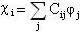(5)

where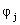describes the different functions that approximate electronic orbitals, and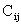are fitting coefficients that are varied in the course of our numerical solution of the Schrödinger equation. In other words, our solution method will involve varying the coefficientsuntil a minimum energy (ground state) solution is found. The energy minimum that is found is not always the absolute global minimum for the molecule, because an molecule can have many local energy minima. A principle known as the variational principle dictates that the lowest energy solution will be closest to the true energy of the molecular system. The larger the basis set size—i.e., the more fitting coefficients we have at our disposal for the solution—the closer to the true ground state energy our solution will be. However, larger basis sets also naturally require longer computational times, because there will be more terms to include in the calculation. An infinite basis set – one that includes innumerable different shapes for the fitting functions – could in principle be used to compute an exact molecular energy. But this calculation would take an infinite amount of time! We typically use the smallest basis set size possible that generates results in reasonably good agreement with experiments.

There are a number of ways to use these basis sets in solving the Schrödinger equation; we will deal only with some of the more commonly used methods here. One of the earliest methods for treating many-electron systems is based on the Hartree-Fock (HF) approximation. The discovery of the HF method was central to the field of quantum chemistry, and it forms the heart of many of the more accurate computational methods today. In the HF method, the energy of each electron is solved for individually, using a modified version of the Schrödinger equation called the Hartree-Fock equation: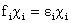(6)

This equation is solved for each electron i, where epsilon (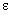) is the energy of the electron analogous to E in the Schrödinger equation, chi (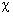) its electronic orbital analogous to, and ( f ) is the so-called Fock operator analogous to H. The Fock operator has the form: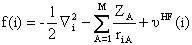(7)

Where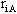is the radial distance be tween the ith electron and the Ath nucleus,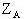is the atomic number of the Ath nucleus, and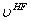is the average potential felt by the ith electron from the presence of the other electrons present in the system.

Solving the complete system of HF equations (as discussed below), one can determine energies for each electron, and sum these together with nuclear nuclear repulsion energies to obtain the total energy of the molecule. The key to the HF approximation is the use of the term, which describes the average electronic charge “felt” by the ith electron; this average is used to compute the effect of electrostatic interactions between electrons on the shapes and energies of the individual orbitals. Taking the average effect of the electrostatic interactions is far less cumbersome to calculate as opposed to calculating the electrostatic effect of the system (the molecule) on each individual electron.

Solution of the HF equations is accomplished by an algorithm known as the self-consistent field (SCF) procedure. The computation basically proceeds as follows:

1. An initial guess for the values of the basis set coefficients,, is made.
2. The values ofare used to construct the electronic orbitals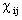through a linear combination of single electron orbitals.
3. Utilizing the electronic orbitals constructed in step 2, which tells you on average the location of the electrons, allows one to determine the average electrostatic interaction () between the electron in question and the remaining electrons in the system. This electrostatic interaction is calcuated for each electron and then averaged to more accurately represent the electron-electron interactions in the molecule.
4. Onceis known, the Hartree-Fock equations can be solved to determine new electronic orbitals and their associated energies.
5. The electronic energies can be summed to calculated the total energy of the system. If the energy is sufficiently converged with respect to the previous SCF cycle, we’re done. If not, return to step 3 and continue to cycle until the energy value converges to a stable (minimum) value.

In many of the programs that are used for these computations, you will be able to watch the progress of the SCF procedure as the calculation progresses.

Although the HF method has produced many valuable results, it turns out that significant errors can be induced by treating repulsive forces between electrons in an average sense, rather than treating them individually. We refer to the difference between the HF energy and the true energy of the system (molecule),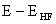, as the correlation energy. There are many methods which attempt to improve the accuracy of computed energies using different methods of accounting for the electron correlation. We list several of these below, along with a very brief description of the basis for each method.

1. Density Functional Theory (DFT). In DFT, the correlation energy is treated as a function of the electronic density, which itself is a function of position. In other words, the correlation can be calculated for a given electronic charge distribution in the molecular system. A number of functionals have been devised for the correlation energy; these functionals vary in their effectiveness for given applications. Treating the correlation energy as a functional of the density greatly improves computational accuracy without a significant cost in terms of computer time. In fact, because other terms in the Schrodinger equation can be treated as functionals of the density, DFT calculations sometimes run faster than HF computations. For this reason, DFT
is widely used for studying complex systems in heterogeneous catalysis, electronic thin films deposition, etc.
2. Perturbation theories. These theories account for the correlation energy in terms of perturbations to the original eigenfunction and eigenvalue solutions. These perturbations are treated as series expansions of the original eigenfunctions. Two of the most popular perturbation methods are referred to as Moller-Plessett calculations, which are commonly based on 2nd-order or 4th-order expansions and referred to as MP2 and MP4, respectively. MP4 is more accurate, but requires longer computation times.
3. Coupled cluster theories. Coupled cluster theories are a method of electronic structure thoery for predicting molecular properties. The basic ideas of CC theories includes cluster expansion, cluster operators and size extensivity. The theory can be furthur categorized into aa formal theory and a pratical theory. The CC equations are quadratic in the ampitudes and must be solved iteratively. CC is correct to the infinite order for single and double excitations and is correct to the 4th order for quadruple excitations.
4. Configuration interaction. Configuration interaction (CI) methods hypothetically can calculate exactly the correlation energy in the limit of an infinite basis set (i.e., an infinite number of fitting parameters). Meaning that the calculation of the total system energy is exact. The price that is paid for this exactness, of course, is an extremely long computation time – full CI is not yet practical for most systems of chemical engineering interest. In CI, the exact wave function is represented as a linear combination of the HF ground state wave function plus excited state wave functions. Similar to perturbation methods, the more excited states that are included in the calculation, the more accurate (and lengthy) the calculation.